• EXCEL移动平均和指数平滑详细描述了excel移动平均法和指数平滑法的操作过程，对于学习非常有帮助。
• 完整的实时深度图平滑代码（像素滤波+加权移动平均） 完整的实时深度图平滑代码（像素滤波+加权移动平均） 完整的实时深度图平滑代码（像素滤波+加权移动平均） 完整的实时深度图平滑代码（像素滤波+加权移动平均
• matlab开发-信号平滑移动平均滤波器。matlab程序演示信号平滑或信号平均的概念
• 平滑移动平均线（SMA） 加权移动平均线（WMA） MACD BOLL歌手乐队（BOLL） 标准差（SD） 最高高价值（HHV） 最低的最低价值（LLV） 安装 $npm i finmath 用法 import { ma , dma , ema , sma , wma , ... • matlab - 信号平滑移动平均滤波 对信号进行平滑操作的重要性不言而喻 1.信号提取 matlab内置了一个这样的数据：某个地方一个月内的温度变化数据，1小时测量一次，所以总数据量是24*31。 可以以这个数据为例子，... matlab - 信号平滑、移动平均滤波 对信号进行平滑操作的重要性不言而喻 1.信号提取 matlab内置了一个这样的数据：某个地方一个月内的温度变化数据，1小时测量一次，所以总数据量是24*31。 可以以这个数据为例子，探究一些数据平滑的方法。该数据如下： clear all close all load bostemp days = (1:31*24)/24; plot(days, tempC) axis tight ylabel('Temp (\circC)') xlabel('Time elapsed from Jan 1, 2011 (days)') title('Logan Airport Dry Bulb Temperature (source: NOAA)')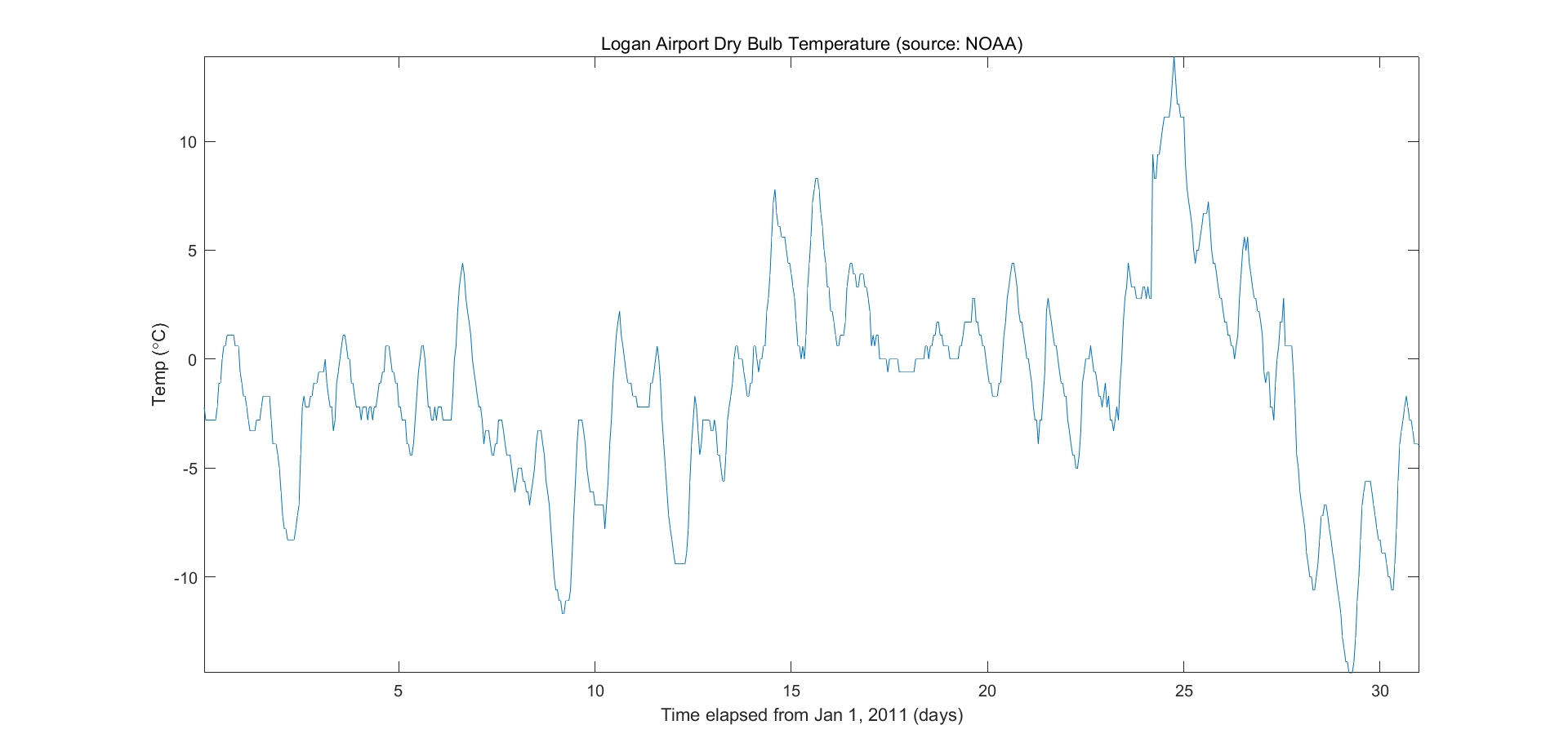上图就是一个月温度的真实变化，凹凸不平。 如果你想看到这一个月内温度的总体变化趋势，而不是关注每天、没小时的变化情况，这时小变化对我们的目的来说就成了噪声，那么就可以将这个数据进行平滑处理，更能反映一个稍大的趋势。移动平均滤波就是一种非常简单有效地平滑方法。 2.均匀移动平均滤波 就不写通用式了，还是举个例子方便理解，有一个数据集x(n)，如果取平均窗长为N=3，那应该有： y ( 0 ) = ( 1 / 3 ) ∗ [ x ( 0 ) ] y(0) = (1/3)*[x(0)] y ( 1 ) = ( 1 / 3 ) ∗ [ x ( 0 ) + x ( 1 ) ] y(1) = (1/3)*[x(0)+x(1)] y ( 2 ) = ( 1 / 3 ) ∗ [ x ( 0 ) + x ( 1 ) + x ( 2 ) ] y(2) = (1/3)*[x(0)+x(1)+x(2)] y ( 3 ) = ( 1 / 3 ) ∗ [ x ( 1 ) + x ( 2 ) + x ( 3 ) ] y(3) = (1/3)*[x(1)+x(2)+x(3)] . . . . . . ...... 下面取N = 24对这个温度数据tempC进行平滑 hoursPerDay = 24; coeff24hMA = ones(1, hoursPerDay)/hoursPerDay; avg24hTempC = filter(coeff24hMA, 1, tempC); plot(days,[tempC avg24hTempC]) legend('Hourly Temp','24 Hour Average (delayed)','location','best') ylabel('Temp (\circC)') xlabel('Time elapsed from Jan 1, 2011 (days)') title('Logan Airport Dry Bulb Temperature (source: NOAA)')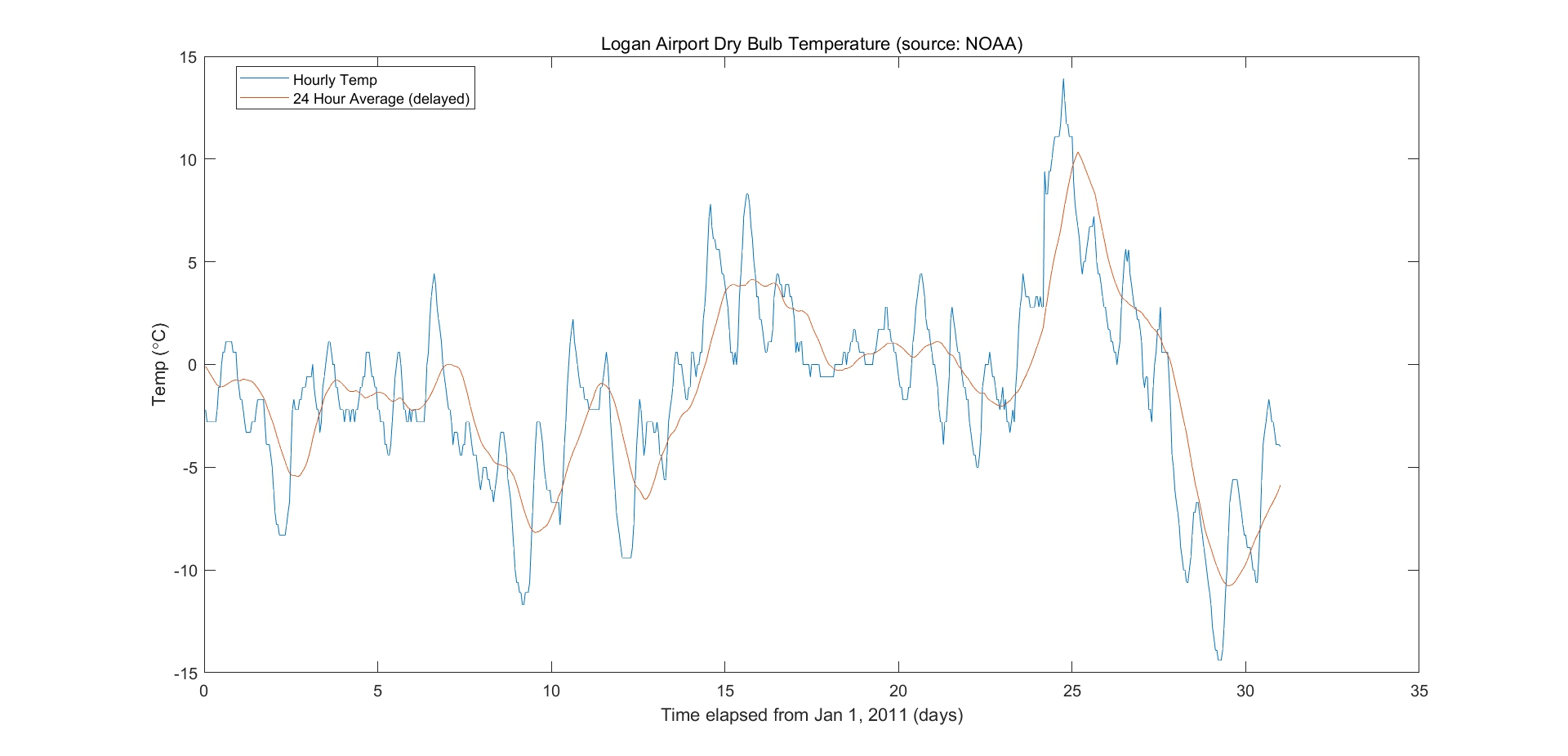输出数据显然平滑了，更能反映一个大的温度变化趋势。当然，平滑后的数据丢失了许多细节。 可以用数字信号处理的观点分析这个平滑过程，移动平均滤波其实就是一个简单的FIR低通滤波器，系统函数h(n)的长度为N，并且对于所有的n都有h(n) = 1/N。这样的系统函数在时域上是个矩形，那么在频域就时sa函数，显然是一个低通滤波器，把高频的量去掉，保留了低频，也就是更大尺度的变化趋势。 细心可以发现，总的来说输出比输入延迟了 (N-1)/2 个单位，这可以用信号与系统的时移性质解释，实际上就是该FIR滤波器的群延时，是个常数。本节重点不在频谱分析，分析就此带过。 移动平均滤波简单还是简单，但是计算量非常大，例如，这里L = 31*24=744个数据，以N =24为平滑窗长，则约需要进行 LN = 1.78万次乘法，L(N-1) = 1.7万次加法。可以使用快速卷积去实现，这样效率更快。 3.均匀移动平均滤波 - 补偿延时 上面分析到一般移动平均滤波延时了（N-1)/2 ，可以进行补偿 fDelay = (length(coeff24hMA)-1)/2; plot(days,tempC, ... days-fDelay/24,avg24hTempC) axis tight legend('Hourly Temp','24 Hour Average','location','best') ylabel('Temp (\circC)') xlabel('Time elapsed from Jan 1, 2011 (days)') title('Logan Airport Dry Bulb Temperature (source: NOAA)')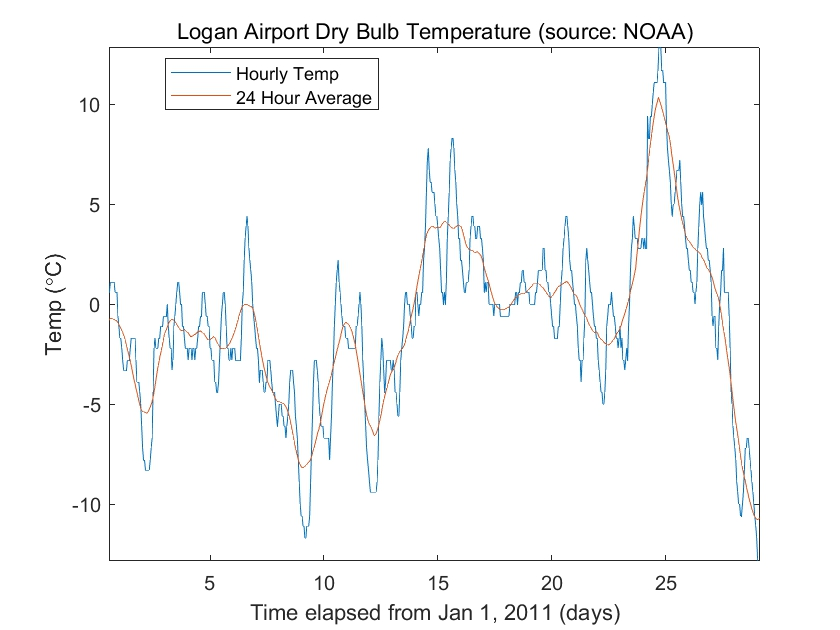这样就得到了我们想要的一个月总体的温度变化情况 4.提取包络线 如果想得到这个月温度的最高温和最低温的变化情况，可以使用提取包络线的办法。 [envHigh, envLow] = envelope(tempC,16,'peak'); envMean = (envHigh+envLow)/2; plot(days,tempC, ... days,envHigh, ... days,envMean, ... days,envLow) axis tight legend('Hourly Temp','High','Mean','Low','location','best') ylabel('Temp (\circC)') xlabel('Time elapsed from Jan 1, 2011 (days)') title('Logan Airport Dry Bulb Temperature (source: NOAA)')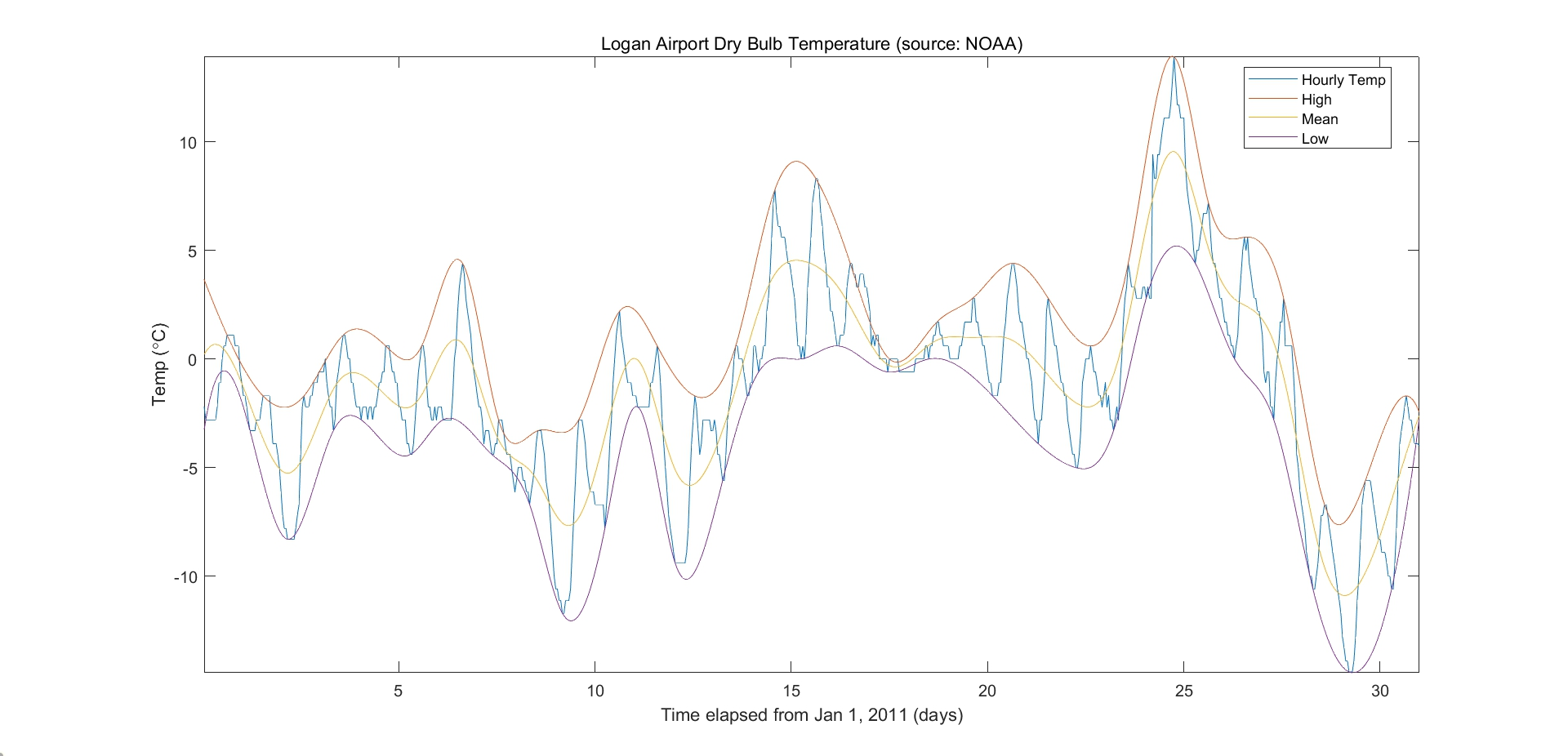5.加权移动平均滤波器 一般的移动平均滤波器的权值时一样的，也可以调整权值。例如，可以使用二项分布的系数作为权值，这时可以用更少的窗长获得更好地平滑效果。这也可以用数字信号处理进行分析，二项分布的系数在时域上比矩形更加接近sa函数，所以对高频分量的滤除效果更好。 h = [1/2 1/2]; binomialCoeff = conv(h,h); for n = 1:4 binomialCoeff = conv(binomialCoeff,h); end figure fDelay = (length(binomialCoeff)-1)/2; binomialMA = filter(binomialCoeff, 1, tempC); plot(days,tempC, ... days-fDelay/24,binomialMA) axis([2.5,6.5,-3,1]) legend('Hourly Temp','Binomial Weighted Average','location','best') ylabel('Temp (\circC)') xlabel('Time elapsed from Jan 1, 2011 (days)') title('Logan Airport Dry Bulb Temperature (source: NOAA)')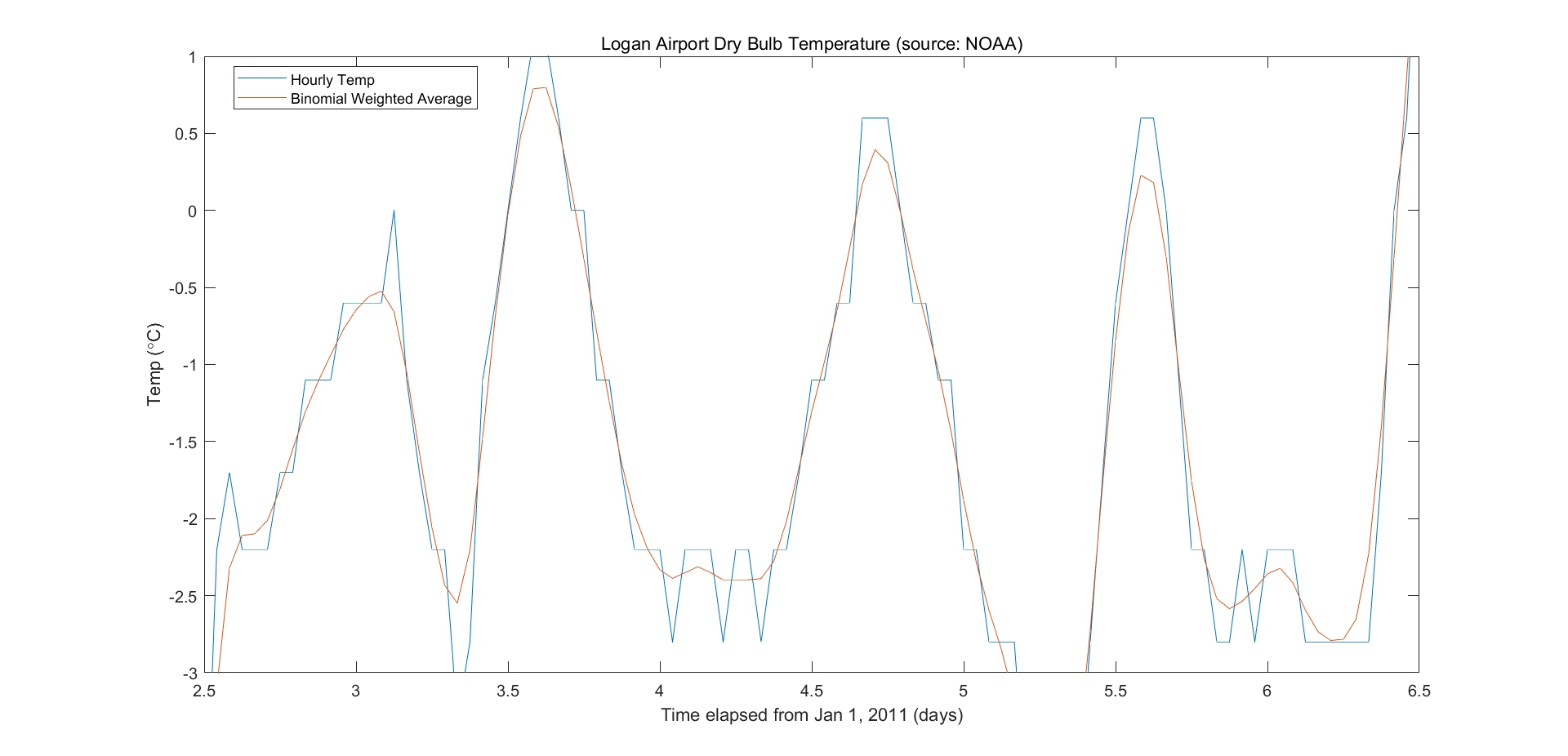6.指数移动平均滤波 指数型移动平均滤波的基本模型是： y ( k ) = a ∗ x ( k ) + ( 1 − a ) ∗ y ( k − 1 ) y(k) = a*x(k) + (1-a)*y(k-1) 其实就是一个简单的IIR滤波器。显然，但a越小，当前输入对输出的影响越小，也就越平滑。可以继续推广到跟高阶。这种滤波器可以用更小的阶数实现比一般移动平均滤波更好地平滑效果。 alpha = 0.20; exponentialMA = filter(alpha, [1 alpha-1], tempC); plot(days,tempC, ... days-fDelay/24,binomialMA, ... days-1/24,exponentialMA) axis([17,20,-1,3]) legend('Hourly Temp', ... 'Binomial Weighted Average', ... 'Exponential Weighted Average','location','best') ylabel('Temp (\circC)') xlabel('Time elapsed from Jan 1, 2011 (days)') title('Logan Airport Dry Bulb Temperature (source: NOAA)')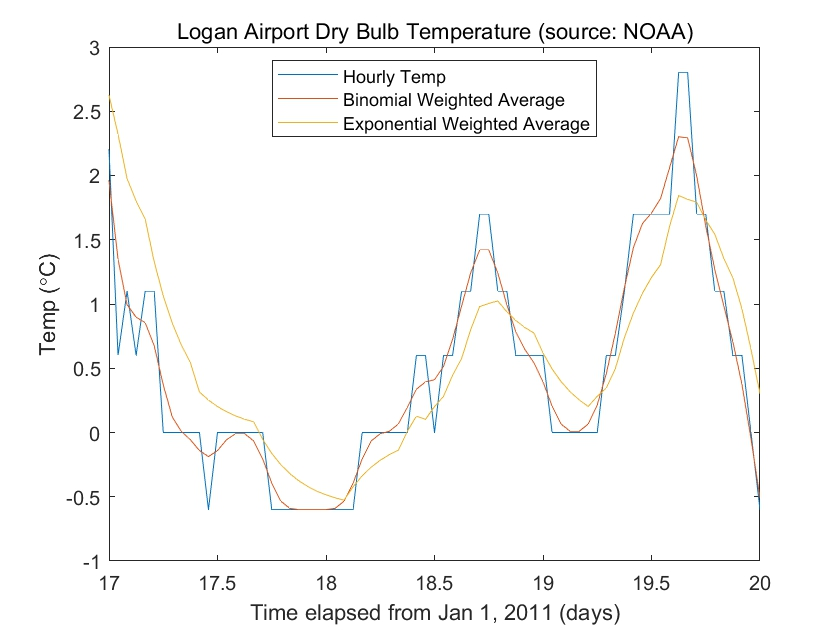7.S-G滤波器 全称叫Savitzky-Golay滤波器，是使用多项式对滑窗内最小二乘法拟合。 cubicMA = sgolayfilt(tempC, 3, 7); quarticMA = sgolayfilt(tempC, 4, 7); quinticMA = sgolayfilt(tempC, 5, 9); plot(days,[tempC cubicMA quarticMA quinticMA]) legend('Hourly Temp','Cubic-Weighted MA', 'Quartic-Weighted MA', ... 'Quintic-Weighted MA','location','southeast') ylabel('Temp (\circC)') xlabel('Time elapsed from Jan 1, 2011 (days)') title('Logan Airport Dry Bulb Temperature (source: NOAA)') axis([3 5 -5 2])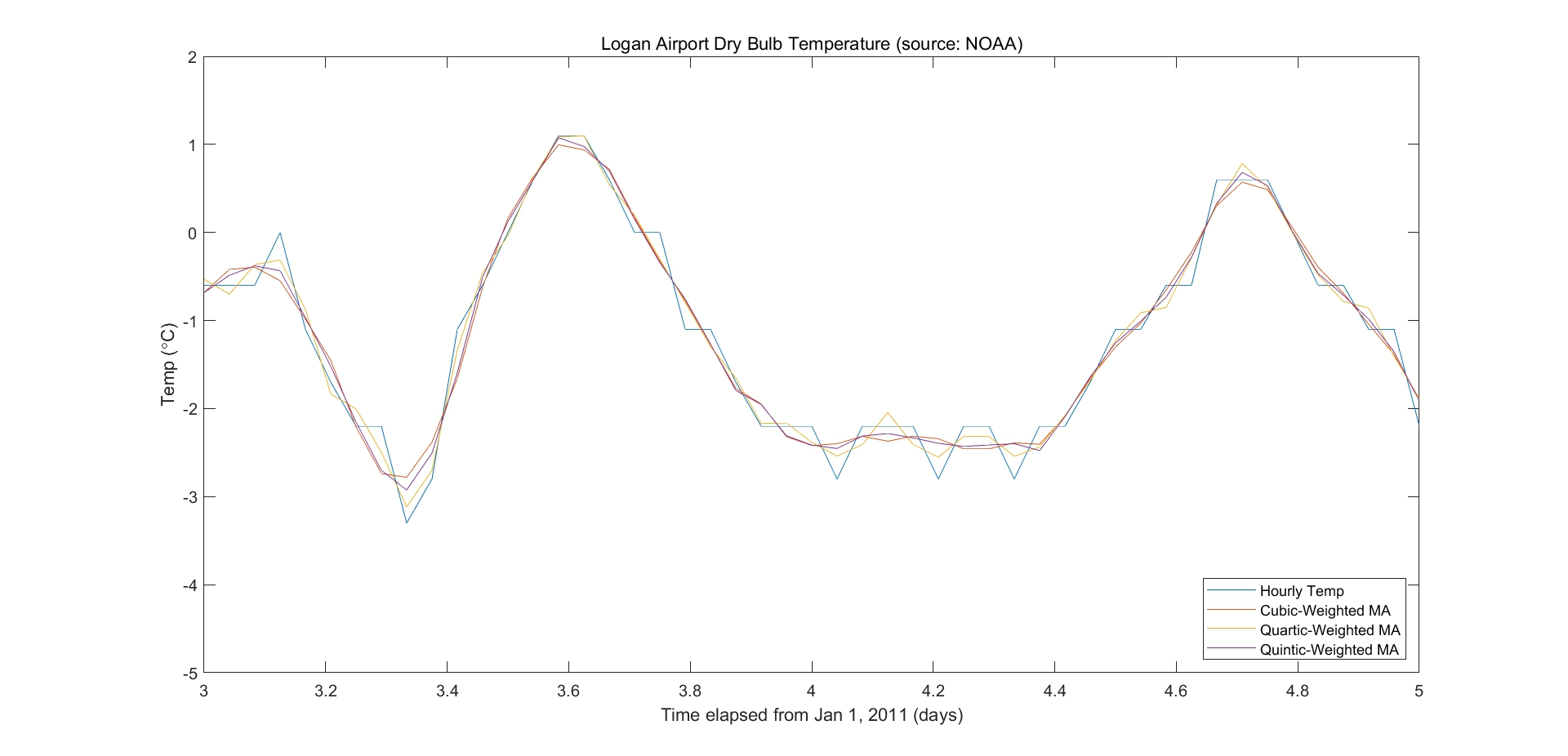8.中值滤波 上面讲的滤波器不管如何，当信号是突变型的时候，就无能为力了，因为上述的滤波器本质上都是加权多项式的移动平均滤波器，例如下面这个时钟信号例子，我们希望把时钟信号的噪声滤除掉。 load clockex yMovingAverage = conv(x,ones(5,1)/5,'same'); ySavitzkyGolay = sgolayfilt(x,3,5); plot(t,x, ... t,yMovingAverage, ... t,ySavitzkyGolay) legend('original signal','moving average','Savitzky-Golay')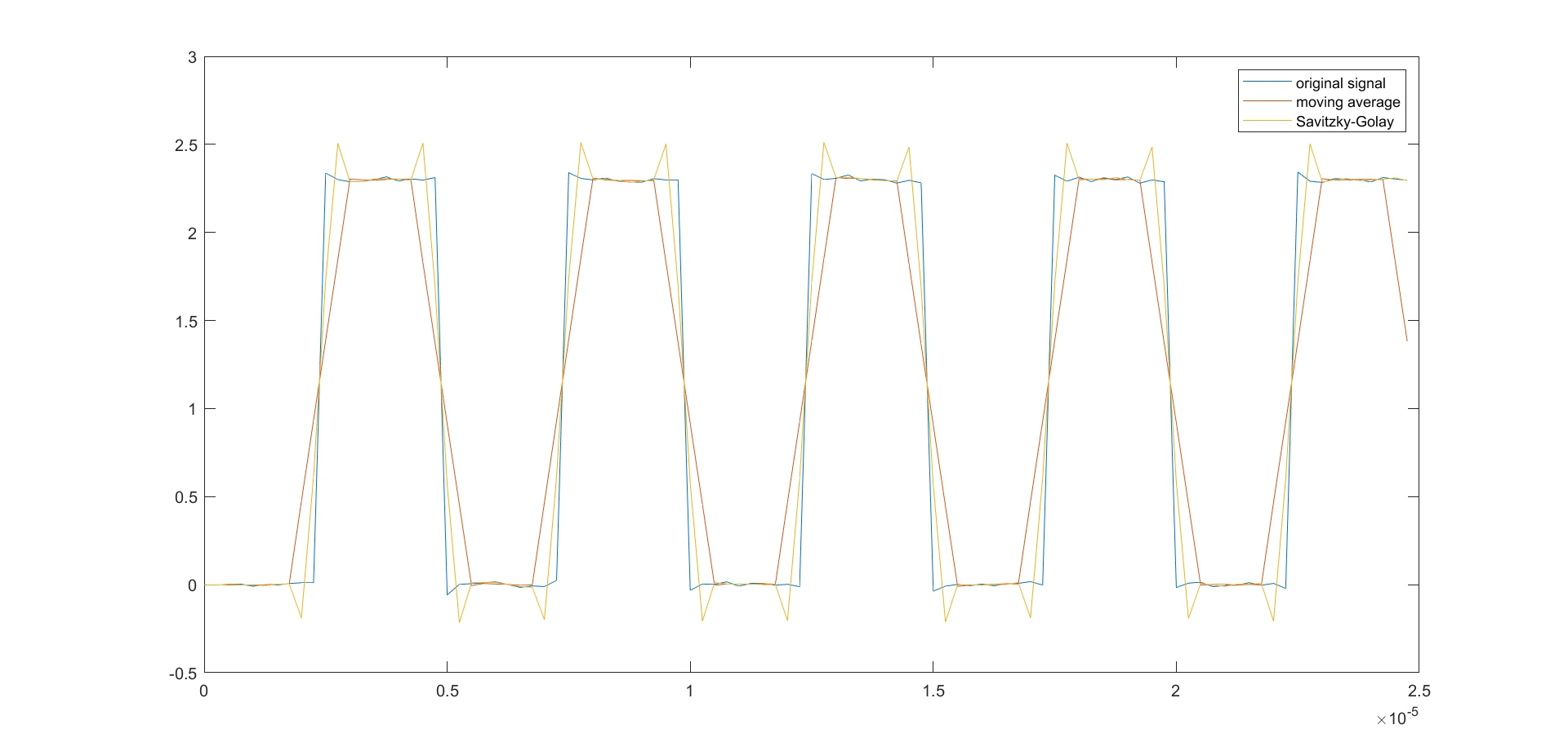可以看到，滤波后的数据要么产生了尖峰，要么变化较慢，都不符合我们的目的。 这时可以采用中值滤波，也可以叫中位值的方法。中值滤波的原理是取一串数据，然后对其排序，取中位数作为当前的数据 中值滤波采用的全是原来的数据，只是进行了排序和取舍。除了这个时钟信号的例子，中值滤波还可以方便地滤除信号中一些尖峰突刺，例如超声波传感器就很常用。 yMedFilt = medfilt1(x,5,'truncate'); plot(t,x, ... t,yMedFilt) legend('original signal','median filter')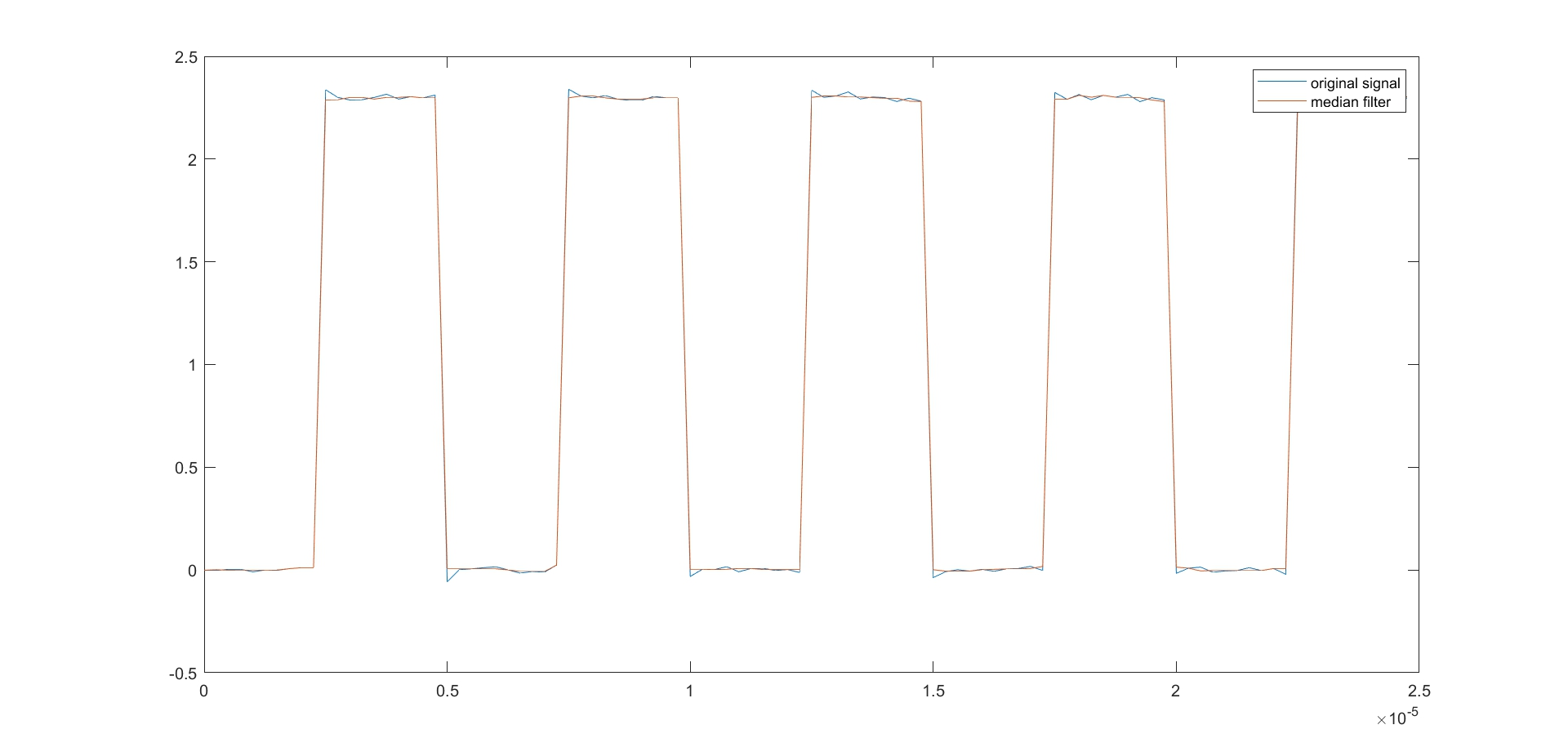可以看到这时候滤波效果非常好 9.Hampel 滤波器 中值滤波滤波也有一些缺点，例如当数据有尖峰毛刺确是可以将其滤除掉，在某些情况下我们希望只将突兀的值滤除掉，而保留原来的信号，但是中值滤波这也将部分有用的信号滤除掉，例如下面这个例子 load train y(1:400:end) = 2.1; plot(y)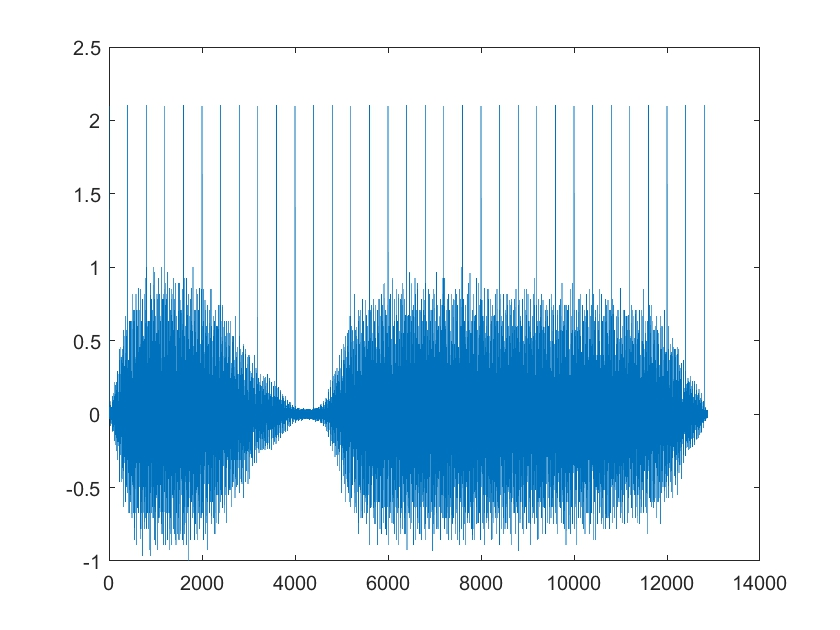我们给原信号加上了一些尖峰点，如果我们采用中值滤波的方法希望将这些尖峰点滤除掉，而保留原信号 hold on plot(medfilt1(y,3)) hold off legend('original signal','filtered signal')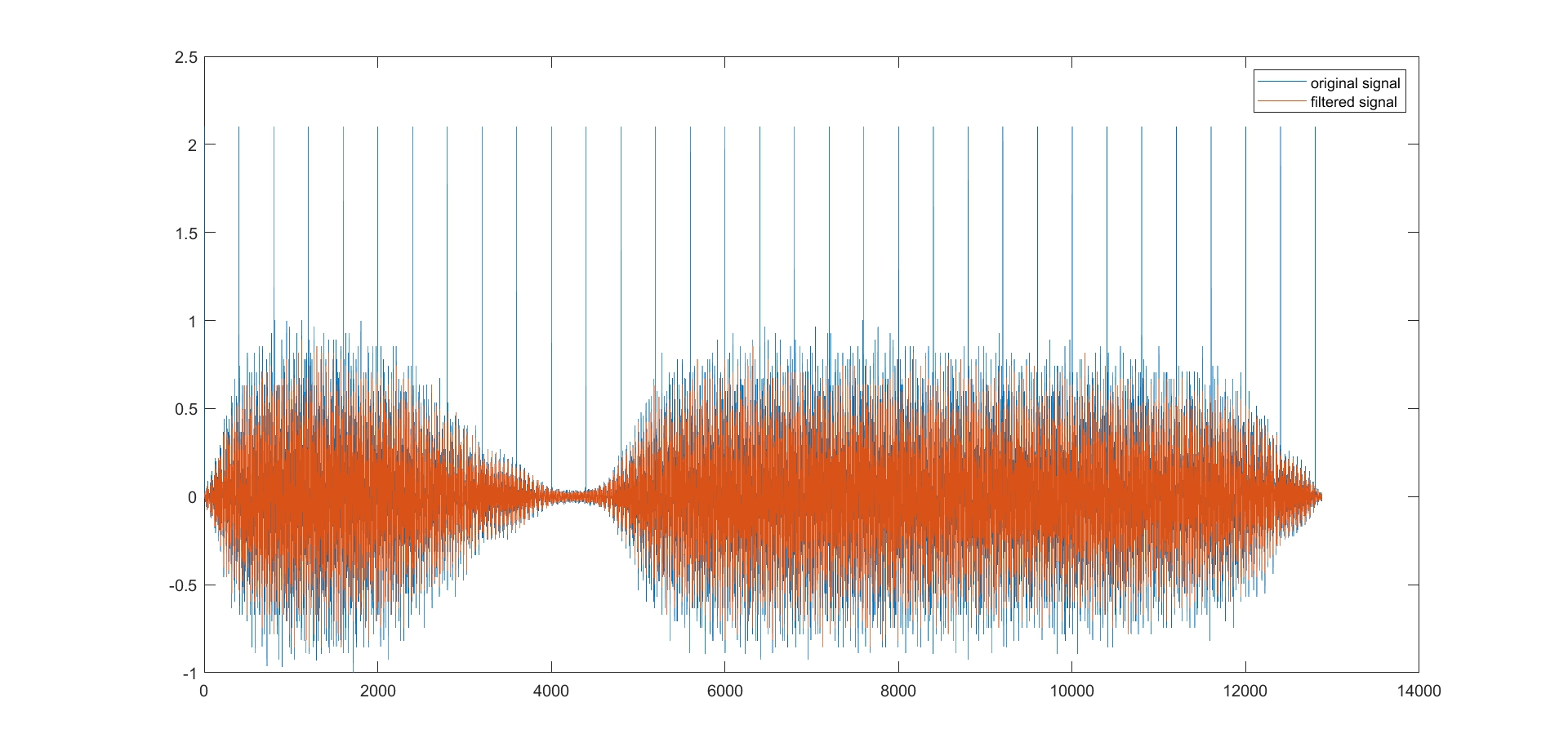利用中值滤波显然可以将外加的尖峰毛刺滤除掉，但是原信号也变了，这不是我们想要看到的 在这种情况下，可以使用Hample滤波器，它与中值滤波器有些相似，但是不是简单地取中位数，也不会随便就把尖峰毛刺扔掉。Hample滤波器会计算附近数据的标准差和均值，以均值为中心，如果该值相差了好几个标准差，才把该值替换成均值与最大能接受的标准差偏差。 hampel(y,13) legend('location','best')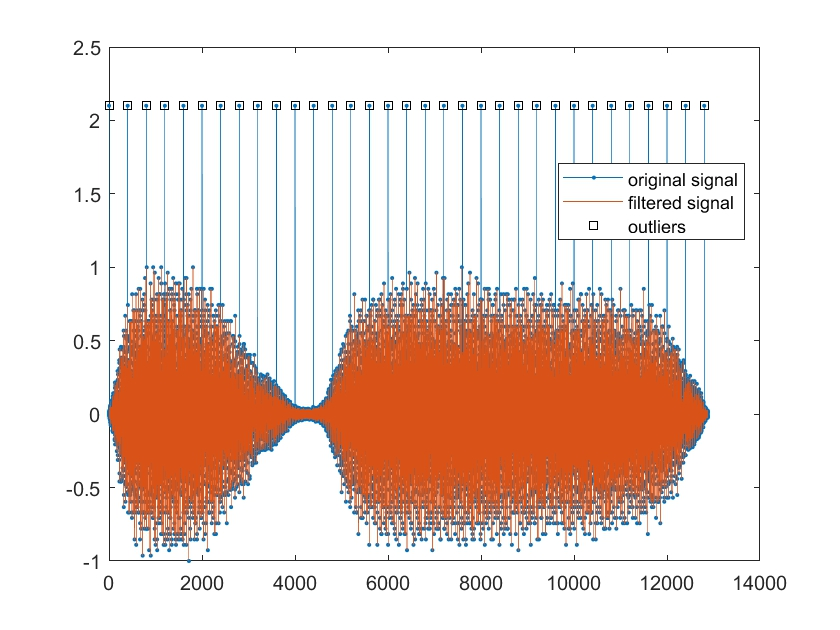可以看到，Hample滤波器也会改变原信号的一些点，但是相比一般中值滤波已经好很多了 10.总结 本节介绍了许多数据平滑的办法。均匀移动平均滤波器是最简单的办法，可以有效消除高频变化，让我们看到数据更大的一个趋势。还可以实际情况改变均匀的权值让效果更好，如二项式拟合和S-G滤波器。指数移动平均滤波让输出数据不断递归，用更低的计算量实现比移动平均滤波器更好地效果。如果需要去除尖峰点，可以使用中值滤波和Hample滤波。 展开全文• 移动平均 用于的实用方法的完整集合，包括： 并且moving-averages还将处理空值。 安装$ npm i moving-averages 用法 import { ma , dma , ema , sma , wma } from 'moving-averages' ma ( [ 1 , 2 , 3 , 4 , 5 ]...JavaScript
• 移动平均法很简单，就是最近的数据预测未来短时间内的数据。有简单移动平均法，真的很简单，就是最近的一组数据，去平均，作为下一时刻的预测： Si=(xi−1+xi−2+...+xi−n)/nS_i = (x_{i-1} + x_{i-2} + ...+ x...
之前介绍了时间序列的基本概念和性质，现在就正式介绍一些处理时间序列的模型方法，第一个是移动平均法。
移动平均法很简单，就是用最近的数据预测未来短时间内的数据。有简单移动平均法，真的很简单，就是用最近的一组数据，去平均，作为下一时刻的预测：

S

i

=

(

x

i

−

1

+

x

i

−

2

+

.

.

.

+

x

i

−

n

)

/

n

S_i = (x_{i-1} + x_{i-2} + ...+ x_{i-n})/n

简单移动平均最大的优点就是通过计算平均可以减小随机波动的影响，当时序数据受到周期变动和随机波动的影响而起伏较大时，通过移动平均就能消除这种影响，进一步分析出它的发展方向与趋势。
简单移动平均的升级版就是加权移动平均，其实就是在各个时刻的数据加上一个权重，考虑了不同时刻的数据对未来的预测的作用的不同，不同于机器学习的模型，这里的权重更多是根据经验法和试算法确定的，个人觉得没有太大研究价值，不再深入讨论。
第二个要介绍的模型是指数平滑模型，它包括一次指数平滑、二次指数平滑、三次指数平滑，当时间序列没有明显趋势变化时，可以使用一次指数平滑进行预测，一次指数平滑的基本公式就是：

S

i

=

α

∗

x

i

+

(

1

−

α

)

S

i

−

1

S_i = \alpha * x_i + (1-\alpha)S_{i-1}

其中S_i是时刻t的预测值，x_i是时刻t的真实值，S_i-1是时刻t-1的预测值，a是平滑常数。一次指数平滑的预测公式是：

x

i

+

k

=

s

i

x_{i+k} = s_i

一次指数平滑可以看成通过结合过去信息和当前信息进行预测，结合的方式就是通过合适的权重进行加权求和，得到的结果是一条直线，所以在序列没有明显趋势的时候才适用（即具有平稳性）。
如果序列具有线性趋势变化，就可以使用二次指数平滑，二次指数平滑的基本公式是：

S

i

=

α

∗

x

i

+

(

1

−

α

)

(

S

i

−

1

+

t

i

−

1

)

S_i= \alpha *x_i + (1-\alpha)(S_{i-1} + t_{i-1})

t

i

=

β

∗

(

s

i

−

s

i

−

1

)

+

(

1

−

β

)

t

i

−

1

t_i = \beta * (s_i - s_{i-1}) + (1-\beta)t_{i-1}

可以看出，二次指数平滑和一次指数平滑相比，加多了一项t_i用于表示趋势，在每个时刻对趋势进行分析，再加入到一次指数平滑中。预测公式是：

x

i

+

h

=

s

i

+

h

∗

t

i

x_{i+h} = s_i + h*t_i

还记得之前提到过，一般的时间序列可表示为：

Z

t

=

T

t

+

S

t

+

X

t

Z_t = T_t + S_t + X_t

其中包含了平稳的序列，趋势变化以及季节性变化，在二次指数平滑的预测公式中，我们看到预测值的等于一次指数平滑的预测值加上表示趋势变化的t_i，如果再继续引入季节性变化呢，就得到三次指数平滑了：

S

i

=

α

∗

(

x

i

−

p

i

−

k

)

+

(

1

−

α

)

(

S

i

−

1

+

t

i

−

1

)

S_i = \alpha *(x_i-p_{i-k}) + (1-\alpha)(S_{i-1} + t_{i-1})

t

i

=

β

∗

(

s

i

−

s

i

−

1

)

+

(

1

−

β

)

t

i

−

1

t_i = \beta * (s_i - s_{i-1}) + (1-\beta)t_{i-1}

p

i

=

γ

∗

(

x

i

−

s

i

)

+

(

1

−

γ

)

p

i

−

k

p_i = \gamma * (x_i - s_i ) + (1-\gamma)p_{i-k}

预测公式为：

x

i

+

h

=

s

i

+

h

∗

t

i

+

p

i

−

k

+

h

x_{i+h} = s_i + h*t_i + p_{i-k+h}

总的来说，指数平滑的实质就是对现在的数据进行加权线性变换，不论是一次二次还是三次，即使是加入趋势和季节变化信息，也是用基本公式表示这些信息，然后汇总起来。

在github写的自然语言处理入门教程，持续更新：NLPBeginner

在github写的机器学习入门教程，持续更新：MachineLearningModels

想浏览更多关于数学、机器学习、深度学习的内容，可浏览本人博客

展开全文时间序列
• 数据集的检查结果移动平均指数平滑 1.实现移动平均 a)一次移动平均，取多个n值，计算标准差； b)二次移动平均，计算参数a,b，进行预测； 代码： # -*- coding=utf-8 -*- # name: nan chen # date: 2021/4/8 14:28 ...


文章目录
1.实现移动平均代码
2.实现指数平滑代码：
3.数据集的检查代码：
结果移动平均指数平滑

1.实现移动平均
a)一次移动平均，取多个n值，计算标准差； b)二次移动平均，计算参数a,b，进行预测；
代码
：
# -*- coding=utf-8 -*-
# name: nan chen
# date: 2021/4/8 14:28

import csv
import matplotlib.pyplot as plt

# 读取数据文件
counts = []
ids = []
ids.append(int(row))
counts.append(int(row))
plt.plot(ids, counts, color="blue", linewidth=1, linestyle=':', marker=',')

length = len(counts)
singemovings = []
pos = 0
min = 1000000
# 一次移动平均
for n in range(2, 201):
singemoving = []
mse_sum = 0
for i in range(n - 1, length):
sum = 0
for j in range(0, n):
sum = sum + (counts[i - j])
singemoving.append(int(sum / n))
singemovings.append(singemoving)
# 求解MSE
for x, y in zip(range(len(counts) - 1, n - 1, -1), range(len(singemoving) - 2, -1, -1)):
mse_sum = mse_sum + ((counts[x] - singemoving[y]) ** 2)
mse = mse_sum / (len(counts) - n)
sq_mse = mse** 0.5
if mse < min:
min = mse
pos = n
print("n=%s 标准差=%s mse=%s" % (n, sq_mse, mse))
# 打印结果
print("n=%s 一次移动平均法的预测值为：%s" % (n, singemoving[len(singemoving) - 1]))
# plt.plot(ids[n - 1:], singemoving, color="red", linewidth=1, linestyle=':', marker=',', label='一次移动平均法')

# 二次移动平均
# 选取mse最小的值计算二次移动平均
n = pos
singemoving = singemovings[n - 2]
twicemoving = []
for i in range(n - 1, len(singemoving)):
sum = 0
for j in range(0, n):
sum = sum + singemoving[i - j]
twicemoving.append(int(sum / n))

# 二次移动平均预测值
a = singemoving[len(singemoving) - 1] * 2 - twicemoving[len(twicemoving) - 1]
b = (2 / (n - 1)) * (singemoving[len(singemoving) - 1] - twicemoving[len(twicemoving) - 1])
x = a + b
print("n=%s 二次移动平均法的预测值为：%s" % (n, x))


2.实现指数平滑
a)一次指数平滑，取多个a值； b)二次指数平滑（可选）；
代码：
# -*- coding=utf-8 -*-
# name: nan chen
# date: 2021/4/9 10:56

import csv
import matplotlib.pyplot as plt

# 读取数据文件
counts = []
ids = []
ids.append(int(row))
counts.append(int(row))

# 一次指数平滑法
s = []
list_a = [2 / (len(counts) + 1), 0.1, 0.2, 0.3, 0.4, 0.5, 0.8, 0.9]
# colors = ["brown", "green", "red", "gray", "yellow"]
# 取初始值为x0
for a in list_a:
s1 = [counts]
for i in range(0, len(counts)):
tmp = a * counts[i] + (1 - a) * s1[i]
s1.append(tmp)
s.append(s1)
for i in range(0, len(list_a)):
s_i = s[i]
print("a=%s 一次指数平滑法的预测值为：%s" % (list_a[i], s_i[len(s_i) - 1]))
# plt.plot(ids, s_i[1:], label='a = %s' % a, color=colors[i], linewidth=1, linestyle=':', marker=',')

# plt.show()

# 二次指数平滑法
twice_s = []
j = 0
for a in list_a:
s2 = [counts]
single_s = s[j]
for i in range(1, len(counts)):
tmp = a * single_s[i] + (1 - a) * s2[i - 1]
s2.append(tmp)
twice_s.append(s2)
j = j + 1

for i in range(0, len(list_a)):
single = s[i]
twice = twice_s[i]
at = 2 * single[len(single) - 1] - twice[len(twice) - 1]
bt = (list_a[i] / 1 - list_a[i]) / (single[len(single) - 1] - twice[len(twice) - 1])
x = at + bt
print("a=%s 二次指数平滑法的预测值为%s" % (list_a[i], x))


3.数据集的检查
代码：
# -*- coding=utf-8 -*-
# name: nan chen
# date: 2021/4/10 9:52
import pandas as pd
import matplotlib.pyplot as plt

# 检查数据集是否存在空缺
total = data.isnull().sum().sort_values(ascending=False)
print(total)

# 绘制散点图观察是否存在偏离值
var = 'ID'
data1 = pd.concat([data['Count'], data[var]], axis=1)
data1.plot.scatter(x=var, y='Count', ylim=(0, 4000), s=1)
plt.show()

# 统计某一列中各个元素值出现的次数
c = data['Count'].value_counts()
print(c)

# 列出数据的偏斜度
ske = data['Count'].skew()
print("Count列的偏斜度%s " % ske)

# 计算count和id的相关系数
cor = data['Count'].corr(data['ID'])
print("Count列与ID列的相关系数为%s " % cor)


结果
移动平均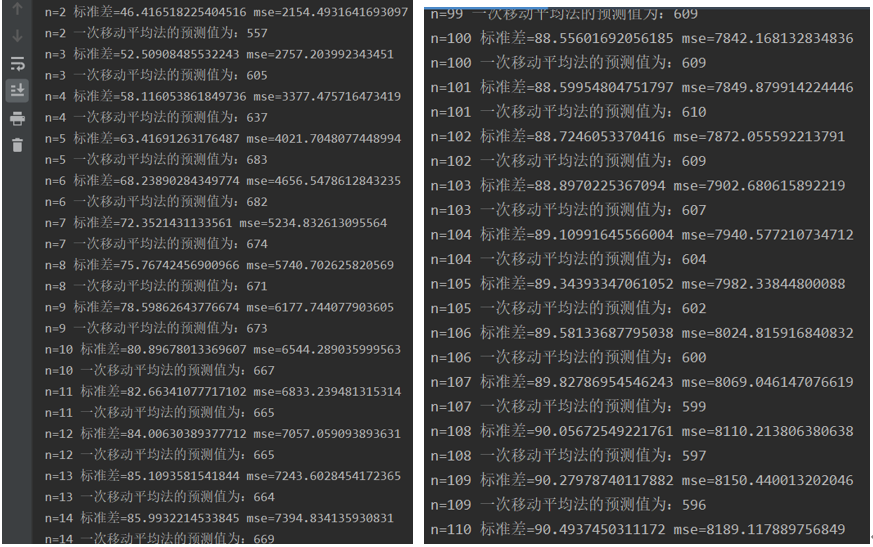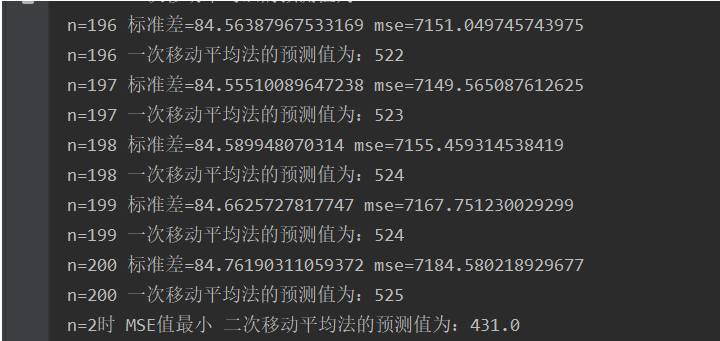指数平滑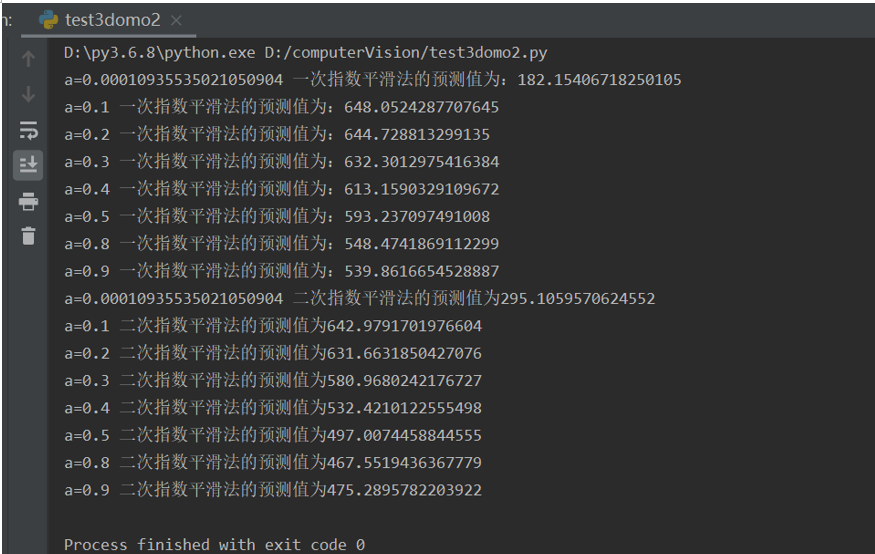展开全文• 移动平均法是一组最近的实际数据值来预测未来一期或几期内公司产品的需求量、公司产能等的一种常用方法。移动平均法适用于即期预测。当产品需求既不快速增长也不快速下降，且不存在季节性因素
____tz_zs
注：本博客中关于概念的解释部分均来自 MBA智库百科

一、移动平均法（Moving average，MA）
移动平均法又称滑动平均法、滑动平均模型法
移动平均法是用一组最近的实际数据值来预测未来一期或几期内公司产品的需求量、公司产能等的一种常用方法。移动平均法适用于即期预测。当产品需求既不快速增长也不快速下降，且不存在季节性因素时，移动平均法能有效地消除预测中的随机波动，是非常有用的。移动平均法根据预测时使用的各元素的权重不同，可以分为：简单移动平均和加权移动平均。
移动平均法是一种简单平滑预测技术，它的基本思想是：根据时间序列资料、逐项推移，依次计算包含一定项数的序时平均值，以反映长期趋势的方法。因此，当时间序列的数值由于受周期变动和随机波动的影响，起伏较大，不易显示出事件的发展趋势时，使用移动平均法可以消除这些因素的影响，显示出事件的发展方向与趋势（即趋势线），然后依趋势线分析预测序列的长期趋势。

（一）简单移动平均法
简单移动平均的各元素的权重都相等。简单的移动平均的计算公式如下：

Ft=(At-1+At-2+At-3+…+At-n)/n

式中，

Ft--对下一期的预测值；

n--移动平均的时期个数；

At-1--前期实际值；

At-2,At-3和At-n分别表示前两期、前三期直至前n期的实际值。

小Demo
.
import matplotlib.pyplot as plt

# 简单移动平均法
def ma(list2):
n = len(list2)
sum = 0
for i in list2:
sum += i
result = sum / n
return result

# 简单移动平均法
listMA = []  # 简单移动平均值的列表
for i in range(n - 1, len(list1)):
# print(i)
list2 = (list1[i - (n - 1):i + 1])
listMA.append(ma(list2))
print("简单移动平均值的列表：{}".format(listMA))
# 最后的移动平均值可做为下一个数的预测
x = listMA[-1]
print("下一个数的预测:{}".format(x))
# 画图
plt.scatter(list(range(len(listMA))), listMA)
plt.show()

if __name__ == '__main__':
list1 = [1, 2, 4, 5, 6, 8, 10, 12, 14, 16, 19, 24, 29]  # 13个
n = 3  # 移动平均期数


.
运行结果：
.
简单移动平均值的列表：[2.3333333333333335, 3.6666666666666665, 5.0, 6.333333333333333, 8.0, 10.0, 12.0, 14.0, 16.333333333333332, 19.666666666666668, 24.0]
下一个数的预测:24.0
.
简单移动平均值散点图：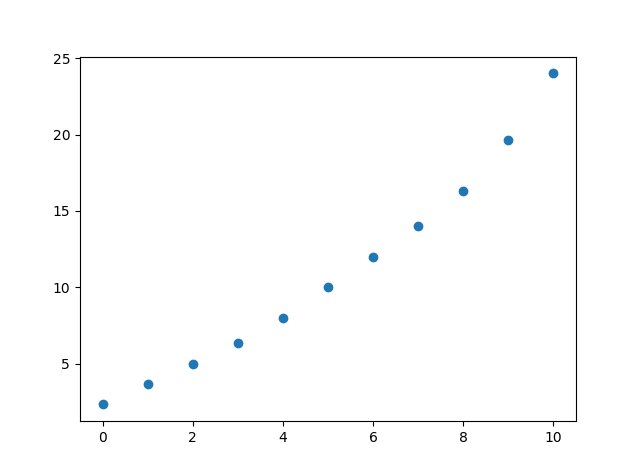（二）加权移动平均法
加权移动平均给固定跨越期限内的每个变量值以不同的权重。其原理是：历史各期产品需求的数据信息对预测未来期内的需求量的作用是不一样的。除了以n为周期的周期性变化外，远离目标期的变量值的影响力相对较低，故应给予较低的权重。 加权移动平均法的计算公式如下：

Ft=w1At-1+w2At-2+w3At-3+…+wnAt-n

式中，

w1--第t-1期实际销售额的权重；

w2--第t-2期实际销售额的权重；

wn--第t-n期实际销售额的权重；

n--预测的时期数；w1+ w2+…+ wn=1

在运用加权平均法时，权重的选择是一个应该注意的问题。经验法和试算法是选择权重的最简单的方法。一般而言，最近期的数据最能预示未来的情况，因而权重应大些。例如，根据前一个月的利润和生产能力比起根据前几个月能更好的估测下个月的利润和生产能力。但是，如果数据是季节性的，则权重也应是季节性的。
小Demo
.
import matplotlib.pyplot as plt

# 加权移动平均法
def wma(list2, w):
n = len(list2)
sum = 0
for i in n:
sum += list2[i] * w[i]
return sum

# 加权移动平均法
w = [0.2, 0.3, 0.5]  # 各期的权重
listWMA = []  # 加权移动平均值的列表
for i in range(n - 1, len(list1)):
# print(i)
list2 = (list1[i - (n - 1):i + 1])
listWMA.append(ma(list2))
print("加权移动平均值的列表：{}".format(listWMA))
# 最后的移动平均值可做为下一个数的预测
x = listWMA[-1]
print("下一个数的预测：{}".format(x))
# 画图
plt.scatter(list(range(len(listWMA))), listWMA)
plt.show()

if __name__ == '__main__':
list1 = [1, 2, 4, 5, 6, 8, 10, 12, 14, 16, 19, 24, 29]  # 13个
n = 3  # 移动平均期数

answer2(list1, n)  # 加权移动平均法
运行结果：
.
加权移动平均值的列表：[2.3333333333333335, 3.6666666666666665, 5.0, 6.333333333333333, 8.0, 10.0, 12.0, 14.0, 16.333333333333332, 19.666666666666668, 24.0]
下一个数的预测：24.0
.
加权移动平均值散点图：.

（三）移动平均法的优缺点
使用移动平均法进行预测能平滑掉需求的突然波动对预测结果的影响。但移动平均法运用时也存在着如下问题：

1、 加大移动平均法的期数（即加大n值）会使平滑波动效果更好，但会使预测值对数据实际变动更不敏感；
2、 移动平均值并不能总是很好地反映出趋势。由于是平均值，预测值总是停留在过去的水平上而无法预计会导致将来更高或更低的波动；
3、 移动平均法要由大量的过去数据的记录。

二、指数平滑法（Exponential Smoothing，ES）
指数平滑法是布朗(Robert G..Brown)所提出，布朗(Robert G..Brown)认为时间序列的态势具有稳定性或规则性，所以时间序列可被合理地顺势推延；他认为最近的过去态势，在某种程度上会持续到最近的未来，所以将较大的权数放在最近的资料。
指数平滑法是生产预测中常用的一种方法。也用于中短期经济发展趋势预测，所有预测方法中，指数平滑是用得最多的一种。简单的全期平均法是对时间数列的过去数据一个不漏地全部加以同等利用；移动平均法则不考虑较远期的数据，并在加权移动平均法中给予近期资料更大的权重；而指数平滑法则兼容了全期平均和移动平均所长，不舍弃过去的数据，但是仅给予逐渐减弱的影响程度，即随着数据的远离，赋予逐渐收敛为零的权数。
也就是说指数平滑法是在移动平均法基础上发展起来的一种时间序列分析预测法，它是通过计算指数平滑值，配合一定的时间序列预测模型对现象的未来进行预测。其原理是任一期的指数平滑值都是本期实际观察值与前一期指数平滑值的加权平均。

（一）指数平滑法的基本公式S_t=a\cdot y_t+(1-a)S_{t-1}

式中，
St--时间t的平滑值；
yt--时间t的实际值；
St − 1--时间t-1的平滑值；
a--平滑常数，其取值范围为[0,1]；
由该公式可知：

1.St是yt和 St − 1的加权算数平均数，随着a取值的大小变化，决定yt和 St − 1对St的影响程度，当a取1时，St = yt；当a取0时，St = St − 1。
2.St具有逐期追溯性质，可探源至St − t + 1为止，包括全部数据。其过程中，平滑常数以指数形式递减，故称之为指数平滑法。指数平滑常数取值至关重要。平滑常数决定了平滑水平以及对预测值与实际结果之间差异的响应速度。平滑常数a越接近于1，远期实际值对本期平滑值影响程度的下降越迅速；平滑常数a越接近于 0，远期实际值对本期平滑值影响程度的下降越缓慢。由此，当时间数列相对平稳时，可取较大的a；当时间数列波动较大时，应取较小的a，以不忽略远期实际值的影响。生产预测中，平滑常数的值取决于产品本身和管理者对良好响应率内涵的理解。
3.尽管St包含有全期数据的影响，但实际计算时，仅需要两个数值，即yt和 St − 1，再加上一个常数a，这就使指数滑动平均具逐期递推性质，从而给预测带来了极大的方便。
4.根据公式S_1=a\cdot y_1+(1-a)S_0，当欲用指数平滑法时才开始收集数据，则不存在y0。无从产生S0，自然无法据指数平滑公式求出S1，指数平滑法定义S1为初始值。初始值的确定也是指数平滑过程的一个重要条件。

如果能够找到y1以前的历史资料，那么，初始值S1的确定是不成问题的。数据较少时可用全期平均、移动平均法；数据较多时，可用最小二乘法。但不能使用指数平滑法本身确定初始值，因为数据必会枯竭。
如果仅有从y1开始的数据，那么确定初始值的方法有：

1）取S1等于y1；

2）待积累若干数据后，取S1等于前面若干数据的简单算术平均数，如：S1=（y1+ y2+y3）/3等等。

（二）指数平滑的预测公式
据平滑次数不同，指数平滑法分为：一次指数平滑法、二次指数平滑法和三次指数平滑法等。
（1） 一次指数平滑预测
当时间数列无明显的趋势变化，可用一次指数平滑预测。其预测公式为：
yt+1'=ayt+(1-a)yt'

式中，

yt+1'--t+1期的预测值，即本期（t期）的平滑值St ；
yt--t期的实际值；
yt'--t期的预测值，即上期的平滑值St-1 。

该公式又可以写作：yt+1'=yt'+a(yt- yt')。可见，下期预测值又是本期预测值与以a为折扣的本期实际值与预测值误差之和。
（2） 二次指数平滑预测二次指数平滑是对一次指数平滑的再平滑。它适用于具线性趋势的时间数列。其预测公式为：

yt+m=(2+am/(1-a))yt'-(1+am/(1-a))yt=(2yt'-yt)+m(yt'-yt) a/(1-a)

式中，
yt= ayt-1'+(1-a)yt-1
显然，二次指数平滑是一直线方程，其截距为：(2yt'-yt)，斜率为：(yt'-yt) a/(1-a),自变量为预测天数。
（3） 三次指数平滑预测
三次指数平滑预测是二次平滑基础上的再平滑。其预测公式是：
yt+m=(3yt'-3yt+yt)+[(6-5a)yt'-(10-8a)yt+(4-3a)yt]*am/2(1-a)2+ (yt'-2yt+yt')*a2m2/2(1-a)2

式中，
yt=ayt-1+(1-a)yt-1

它们的基本思想都是：预测值是以前观测值的加权和，且对不同的数据给予不同的权，新数据给较大的权，旧数据给较小的权。

（三）指数平滑法的趋势调整一段时间内收集到的数据所呈现的上升或下降趋势将导致指数预测滞后于实际需求。通过趋势调整，添加趋势修正值，可以在一定程度上改进指数平滑预测结果。

调整后的指数平滑法的公式为：

包含趋势预测(YITt)=新预测（Yt）+趋势校正（Tt）

进行趋势调整的指数平滑预测有三个步骤：

1、 利用前面介绍的方法计算第t期的简单指数平滑预测（Yt）；
2、 计算趋势。其公式为： Tt=(1-b)Tt-1+b(Yt-Yt-1)其中，

Tt=第t期经过平滑的趋势；

Tt-1=第t期上期经过平滑的趋势；

b=选择的趋势平滑系数；

Yt=对第t期简单指数平滑预测；

Yt-1=对第t期上期简单指数平滑预测。

3、计算趋势调整后的指数平滑预测值（YITt）。计算公式为：YITt=Yt+Tt。

.
import matplotlib.pyplot as plt

# 一次指数平滑预测
def es1(list3, t, a):
if t == 0:
return list3  # 初始的平滑值取实际值

return a * list3[t - 1] + (1 - a) * es1(list3, t - 1, a)  # 递归调用 t-1 → 12

# 二次指数平滑预测
def es2(list3, t, a):
if t == 0:
return list3

return (a * es2(list3, t - 1, a) + (1 - a) * list3[t - 1])

# 指数平滑法
a = 0.8  # 平滑常数
listES = []  # 指数平滑值的列表
for i in range(len(list2)):
if i == 0:
listES.append(list2[i])
continue
s = a * list2[i] + (1 - a) * listES[-1]
listES.append(s)
print("指数平滑值的列表：{}".format(listES))

# 画图
plt.scatter(list(range(len(listES))), listES)
plt.show()

# 一次指数平滑预测
t = len(list2)  # 预测的时期 13
x = es1(list2, t, a)
print("下一个数的一次指数平滑预测：{}".format(x))

# 二次指数平滑预测
m = 3  # 预测的值为之后的第m个
yt = es2(list2, t - 1, a)
ytm = listES[t - 2]
esm = ((2 * ytm - yt) + m * (ytm - yt) * a / (1 - a))
print("之后的第{}个数的二次指数平滑预测：{}".format(m, esm))

if __name__ == '__main__':
list1 = [1, 2, 4, 5, 6, 8, 10, 12, 14, 16, 19, 24, 29]  # 13个
n = 3  # 移动平均期数

.
运行结果：
.
指数平滑值的列表：[1, 1.8, 3.56, 4.712, 5.742400000000001, 7.5484800000000005, 9.509696, 11.5019392, 13.50038784, 15.500077568, 18.300015513600002, 22.860003102720004, 27.772000620544002]
下一个数的一次指数平滑预测：27.772000620544002
之后的第3个数的二次指数平滑预测：137.5839514214401
.
指数平滑值的散点图：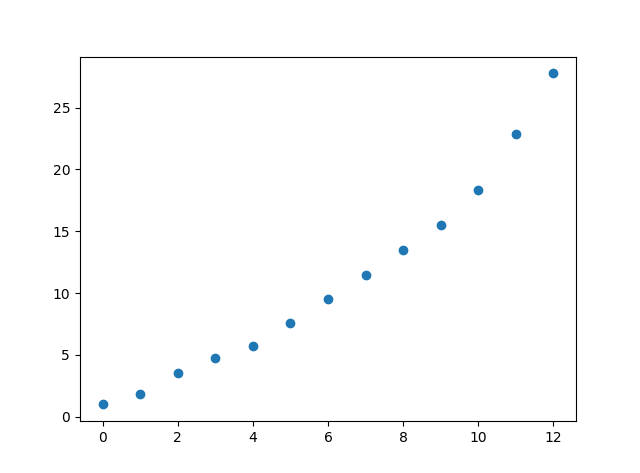.
展开全文MA ES
• 移动平均是过滤受噪声影响的信号的基本概念。 在这个程序中实现了相同的概念，平均每帧 3 个样本matlab
• 1. 实现移动平均； a) 一次移动平均，取多个n值，计算标准差； b) 二次移动平均，计算参数a,b，进行预测； 2. 实现指数平滑。 a) 一次指数平滑，取多个a值； b) 二次指数平滑（可选）；python
• 指数平滑法 原数数据如下： 点击数据——数据分析 选择指数平滑 最一次平滑 由于我们选择的区域是B1：B22，第一个单元格“钢产量”，被当做标志，所以我们应该勾选标志。当我们勾选了标志后，列中的第...
• 从上图看到，3期、7期简单移动平均都对原对数序列作了平滑，7期简单移动平均平滑程度更高，证明了简单移动平均的期数越多越平滑。同时，简单移动平均 消除 了一定的 波动 ，甚至 消除 了一些 季节变动 影响，这...Eviews
• 版权所有：2018 - Pertamina 大学地球物理工程网址： ... Maulud, Ilham (2018)：使用移动平均线平滑一维。 无花果。 https://doi.org/10.6084/m9.figshare.5946844.v1matlab
• ＭＡＣＤ是根据移动平均线较易掌握趋势变动的方向之优点所发展出来的，它是利用二条不同速度（一条变动的速率快──短期的移动平均线，另一条较慢──长期的移动平均线）的指数平滑移动平均线来计算二者之间的差离...
• a) 一次移动平均，取多个n值，计算标准差； b) 二次移动平均，计算参数a,b，进行预测； 实现指数平滑。 a) 一次指数平滑，取多个a值； b) 二次指数平滑（可选）； 二、实验平台 Python 3.7 三、实验内容与结果：...python 算法
• 求当日价格X的N日指数平滑移动平均，在股票公式中一般表达为：EMA（X，N），其中X为当日收盘价，N为天数。它真正的公式表达是：当日指数平均值=平滑系数*（当日指数值-昨日指数平均值）+昨日指数平均值；平滑系数=2/...
• 一、移动平均法（Moving average , MA） 移动平均法又称滑动平均法、滑动平均模型。 用处：一组最近的实际数据值->...分类：简单移动平均 和 加权移动平均 思想：根据时间序列资料，逐项推移...
• MOVING 将计算 n 阶移动平均线（最好将其视为奇数） 用法：y=moving(x,n[,fun]) 其中 x 是要平滑的输入向量（或矩阵）。 m 是要平均的点数（最好的奇数，但偶数有效） y是与x长度相同的输出向量fun（可选）是自定义...matlab
• Java和H2O-3中实现的指数平滑移动平均模型 纯Java和中指数平滑移动平均的实现 Java实现： 统计计算： 转换算法： 移动平均算法： 平稳性测试： 预测算法： DoubleExponentialSmoothing.java ...
• 它计算蒂尔森移动平均线。 用户可以更改平滑扫描和体积因子等参数。matlab
• （1）简单移动平均 移动平均在TTR包中  简单移动平均 SMA(x, n = 10, ...) 指数移动平均 EMA(x, n = 10, wilder = FALSE, ratio = NULL, ...)    For EMA, wilder=FALSE (the default) uses an exponential ...
• 移动平均 import numpy as np def Move_average(list, n): list = np.convolve(lis, np.ones(n) / n, mode='valid') print("移动平均: ", list) return list def Std(list): std = np.std(list) pri...python 数据分析
• 移动平均移动平均法是一组最近的实际数据来预测未来一期或几期内目标值的常用方法。移动平均法适用于即期预测，当时间序列不会快速变化，且不存在季节性因素的时候，移动平均法能有效地消除预测中的...python 机器学习 人工智能 深度学习 数据分析
• 顾名思义，移动窗口平均平滑的意思就是平滑窗口在数据上移动平均，从而对数据进行去噪。上一节我们讲的标准化是对全体样本数据的移动、缩放，从而消除各个样本数据间的差异。而移动窗口平均平滑是对单个样本数据...
• 移动平均滤波器是一种简单的低通 FIR（有限脉冲响应）滤波器，通常用于平滑数据中的随机变化。 .matlab
• 此示例显示如何使用移动平均滤波器和重采样来隔离每小时温度读数的时间周期分量的影响，以及从开环电压测量中消除不需要的线路噪声。 该示例还显示了如何使用Hampel过滤器来移除大异常值。 动机 平滑是我们如何...matlab...